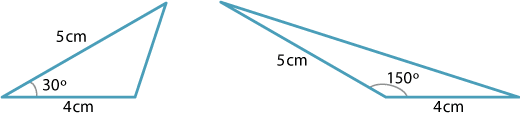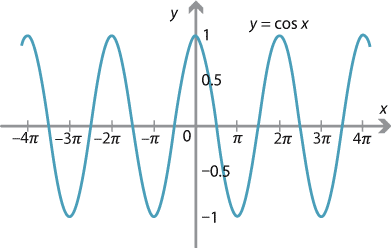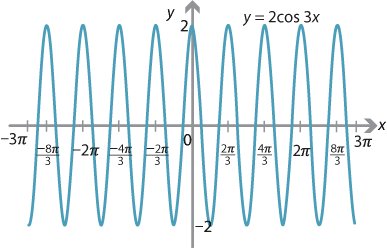## Answers to exercises

##### Exercise 1
Coordinates of $$P$$ $$\theta$$ $$\sin\theta$$ $$\cos\theta$$ $$\tan\theta$$
(1,0) $$0^\circ$$ 0 1 0
(0,1) $$90^\circ$$ 1 0 undefined
(−1,0) $$180^\circ$$ 0 −1 0
(0,−1) $$270^\circ$$ −1 0 undefined
(1,0) $$360^\circ$$ 0 1 0
##### Exercise 2
1. \begin{aligned}[t] \sin 330^\circ &= -\sin 30^\circ = -\dfrac{1}{2}\\ \cos 330^\circ &= \cos 30^\circ = \dfrac{\sqrt{3}}{2}\\ \tan 330^\circ &= -\tan 30^\circ = -\dfrac{1}{\sqrt{3}} \end{aligned}
2. If $$\theta$$ is in the fourth quadrant, the related angle is $$360^\circ-\theta$$.
##### Exercise 3
1. $$\sin 210^\circ = -\dfrac{1}{2}$$
2. $$\cos 315^\circ = \dfrac{1}{\sqrt{2}}$$
3. $$\tan 150^\circ = -\dfrac{1}{\sqrt{3}}$$
##### Exercise 4
1. In $$\triangle BCP$$, we have $$h = a\,\sin B$$. In $$\triangle ACP$$, we have $$h = b\,\sin A$$.
2. Hence $$a\,\sin B = b\,\sin A \ \implies \ \dfrac{a}{\sin A} = \dfrac{b}{\sin B}$$.
3. In $$\triangle BCM$$, we have $$h = a\,\sin B$$. In $$\triangle ACM$$, we have $$h = b\,\sin(180^\circ - A) = b\,\sin A$$.
4. Again it follows that $$\dfrac{a}{\sin A} = \dfrac{b}{\sin B}$$.
##### Exercise 5

Using $$A = \dfrac{1}{2} ab\,\sin \theta$$, we have

\begin{align*} 5 = \dfrac{1}{2}\times 5\times 4 \times \sin \theta \ &\implies \ \sin \theta = \dfrac{1}{2}\\ \ &\implies \ \theta = 30^\circ, 150^\circ. \end{align*}Detailed description of diagram

##### Exercise 6
$$\dfrac{1}{2}ac\,\sin B = \dfrac{1}{2}bc\,\sin A \ \implies \ a\,\sin B = b\,\sin A \ \implies \ \dfrac{a}{\sin A} = \dfrac{b}{\sin B}$$
##### Exercise 7
$$\text{LHS} = \dfrac{1}{\sec\theta + \tan \theta} = \dfrac{1}{\dfrac{1}{\cos\theta} + \dfrac{\sin\theta}{\cos\theta}} = \dfrac{\cos\theta}{1+\sin\theta} = \text{RHS}$$
##### Exercise 8

The double angle formula for cosine gives $$\cos 30^\circ = 1 - 2\sin^2 15^\circ$$. So

\begin{align*} \sin^2 15^\circ &= \dfrac{1}{2}\bigl(1 - \cos 30^\circ\bigr)\\ &= \dfrac{1}{2}\Bigl(1 - \dfrac{\sqrt 3}{2}\Bigr)\\ &= \dfrac{1}{4}\bigl(2 - \sqrt 3\bigr). \end{align*}

Hence $$\sin 15^\circ = \dfrac{1}{2}\sqrt{2 - \sqrt{3}}$$. (Take the positive square root, as $$\sin15^\circ$$ is positive.)

An alternative method is to start from $$\sin 30^\circ = 2\sin 15^\circ\cos 15^\circ$$. Proceed by squaring both sides of the equation and using the Pythagorean identity.

##### Exercise 9

Using the double angle formulas, we have

$\tan 2\theta = \dfrac{\sin2\theta}{\cos 2\theta} = \dfrac{2\sin\theta\,\cos\theta}{\cos^2\theta - \sin^2\theta}.$

If we assume that $$\tan \theta$$ is defined, then we can divide the numerator and denominator by $$\cos^2\theta$$ to obtain

$\tan 2\theta = \dfrac{2\,\dfrac{\sin\theta}{\cos\theta}}{1-\dfrac{\sin^2\theta}{\cos^2\theta}} = \dfrac{2\tan\theta}{1-\tan^2\theta}.$

Note that $$\tan 2\theta$$ is defined if and only if $$\cos 2\theta = \cos^2\theta - \sin^2\theta \ne 0$$, in which case $$\tan \theta$$ cannot equal $$\pm 1$$.

##### Exercise 10
\begin{align*} \sin(A+B) &= \cos\bigl(90^\circ -(A+B)\bigr)\\ &= \cos\bigl( (90^\circ -A)-B\bigr)\\ &= \cos(90^\circ -A)\,\cos B + \sin(90^\circ - A)\,\sin B\\ &= \sin A\,\cos B + \cos A\,\sin B. \end{align*}

Replacing $$B$$ with $$-B$$ gives the remaining formula.

##### Exercise 11
1. These follow easily by considering the triangles $$BCD$$ and $$ACD$$.
2. Area of $$\triangle ABC$$ $$=$$ area of $$\triangle BCD$$ $$+$$ area of $$\triangle ACD$$.
3. So $$\dfrac{1}{2} ab\,\sin(\alpha + \beta) = \dfrac{1}{2} ay\,\sin \alpha + \dfrac{1}{2} by\,\sin \beta$$.
4. Substitute $$y = b\cos\beta$$ into the first term on the right-hand side of the equation from part (b) and $$y=a\cos\alpha$$ into the second term. Then divide both sides by $$\dfrac{1}{2}ab$$ to obtain the sine expansion.
##### Exercise 12
$$\tan 15^\circ = \tan(45^\circ - 30^\circ) = \dfrac{1-\frac{1}{\sqrt{3}}}{1+\frac{1}{\sqrt{3}}} = \dfrac{\sqrt{3}-1}{\sqrt{3}+1}$$ (By multiplying both the numerator and the denominator by $$\sqrt{3} - 1$$, this answer can be simplified to $$\tan 15^\circ = 2 - \sqrt{3}$$.)
##### Exercise 13

Put $$\theta = 67\frac{1}{2}^\circ$$ into the double angle formula:

\begin{align*} \tan 135^\circ = \dfrac{2t}{1-t^2} \ &\implies \ -1 = \dfrac{2t}{1-t^2}\\ \ &\implies \ t^2 - 2t - 1 = 0\\ \ &\implies \ t = 1 \pm \sqrt{2}. \end{align*}

Hence, $$\tan 67\frac{1}{2}^\circ =1+\sqrt{2}$$.

(Here we take the positive square root, since $$\tan 67\frac{1}{2}^\circ$$ is positive.)
##### Exercise 14

With $$m_2=2$$ and $$\gamma = 45^\circ$$, we have

$1 = \Biggl|\dfrac{m-2}{1+2m}\Biggr|.$

Hence $$m-2 = 2m+1$$ or $$m-2 = -(2m+1)$$, giving $$m = -3$$ and $$m=\dfrac{1}{3}$$.

The two lines obtained are perpendicular.

##### Exercise 15

Since the angles subtended at the centre of the hexagon are each $$60^\circ$$, we can divide the inner and outer hexagons into equilateral triangles. The side length of the inner triangles is $$r$$. After applying Pythagoras' theorem, we find that the side length of each triangle in the outer hexagon is $$\dfrac{2}{\sqrt{3}} r$$. Hence, comparing perimeters,

$6r \leq 2\pi r \leq 6 \times \dfrac{2}{\sqrt{3}}r.$

Dividing by $$2r$$ and simplifying gives the desired result.

##### Exercise 16##### Exercise 17##### Exercise 18
\begin{align*} \sin 3\theta &= \sin(2\theta +\theta)\\ &= \sin 2\theta\,\cos \theta + \cos 2\theta\,\sin \theta\\ &= 2\sin\theta\,\cos^2\theta + \bigl(\cos^2\theta-\sin^2\theta\bigr)\,\sin\theta\\ &= 2\sin\theta\,\bigl(1-\sin^2\theta\bigr)+\bigl(1-2\sin^2\theta\bigr)\,\sin\theta\\ &= 3\sin\theta - 4\sin^3\theta \end{align*}
##### Exercise 19

There are a number of ways to do this problem. We could begin by factoring out 5, but we will do it as follows:

$A\sin(x + \alpha) = A\bigl(\sin x\,\cos \alpha + \cos x\,\sin \alpha\bigr) = 3\cos x - 4\sin x.$

Since this is an identity in $$x$$, we can equate coefficients and write

$A\sin \alpha = 3 \qquad \text{and} \qquad A\cos \alpha = -4.$

Squaring and adding these equations gives $$A=5$$, and hence $$\sin \alpha = \dfrac{3}{5}$$ and $$\cos \alpha = -\dfrac{4}{5}$$. So we can take $$\alpha$$ as an angle in the second quadrant and the calculator gives $$\alpha \approx 143.1^\circ$$. Hence $$3\cos x - 4\sin x = 5\sin(x+\alpha)$$, with $$\alpha \approx 143.1^\circ$$.

##### Exercise 20

The double angle formula for cosine gives $$\cos \theta = \cos^2 {\dfrac{\theta}{2}} - \sin^2 {\dfrac{\theta}{2}}$$. So, using the triangle arising from $$t= \tan {\dfrac{\theta}{2}}$$, we have

$\cos \theta = \dfrac{1}{1+t^2} - \dfrac{t^2}{1+t^2} = \dfrac{1-t^2}{1+t^2}.$
##### Exercise 21

Let $$\alpha = \dfrac\theta2$$ and $$t = \tan \alpha$$. Then

$\dfrac{2t}{1 + t^2} = \dfrac{\dfrac{2\sin\alpha}{\cos\alpha}}{1 + \dfrac{\sin^2\alpha}{\cos^2\alpha}} = \dfrac{2\sin\alpha\,\cos\alpha}{\cos^2\alpha + \sin^2\alpha} = 2\sin\alpha\,\cos\alpha = \sin2\alpha = \sin\theta,$ using the Pythagorean identity and the double angle formula for sine.